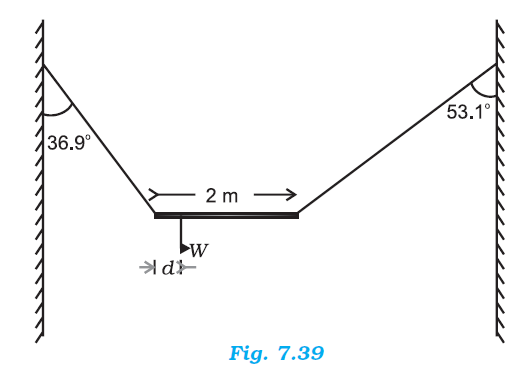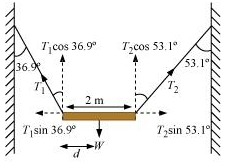## Pages

### System of particles and Rotational motion NCERT Solutions Class 11 Physics - Solved Exercise Question 7.8

Question 7.8:
A non-uniform bar of weight W is suspended at rest by two strings of negligible weight as shown in Fig.7.39. The angles made by the strings with the vertical are 36.9° and 53.1° respectively. The bar is 2 m long. Calculate the distance d of the centre of gravity of the bar from its left end.Solution:
The free body diagram of the bar is shown in the following figure.Length of the bar, l = 2 m
T1 and T2 are the tensions produced in the left and right strings respectively.

At translational equilibrium, we have:
T1 Sin 36.90 = T2 Sin 53.10
T1 / T2 = 4 / 3
T1 = (4/3) T2
For rotational equilibrium, on taking the torque about the centre of gravity, we have:
T1 (Cos 36.9) X d = T2 Cos 53.1 (2 - d)
T1 X 0.800 d = T2 X 0.600 (2 - d)
(4/3) X T2 X 0.800d = T2 (0.600 X 2 - 0.600d)
1.067d + 0.6d = 1.2
∴ d = 1.2 / 1.67
= 0.72 m
Hence, the C.G. (centre of gravity) of the given bar lies 0.72 m from its left end.